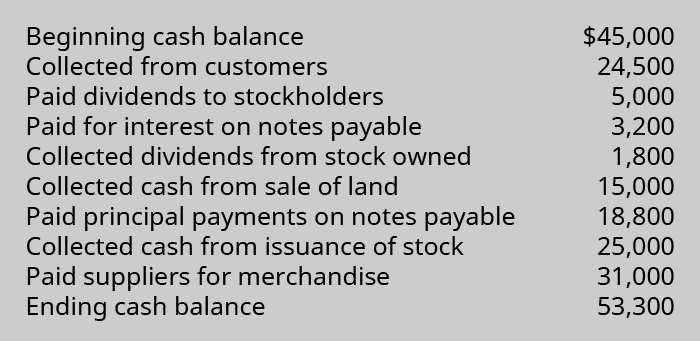This book is archived and will be removed July 6, 2022. Please use the updated version.

Statement of Cash Flows

# 100 Appendix: Prepare a Completed Statement of Cash Flows Using the Direct Method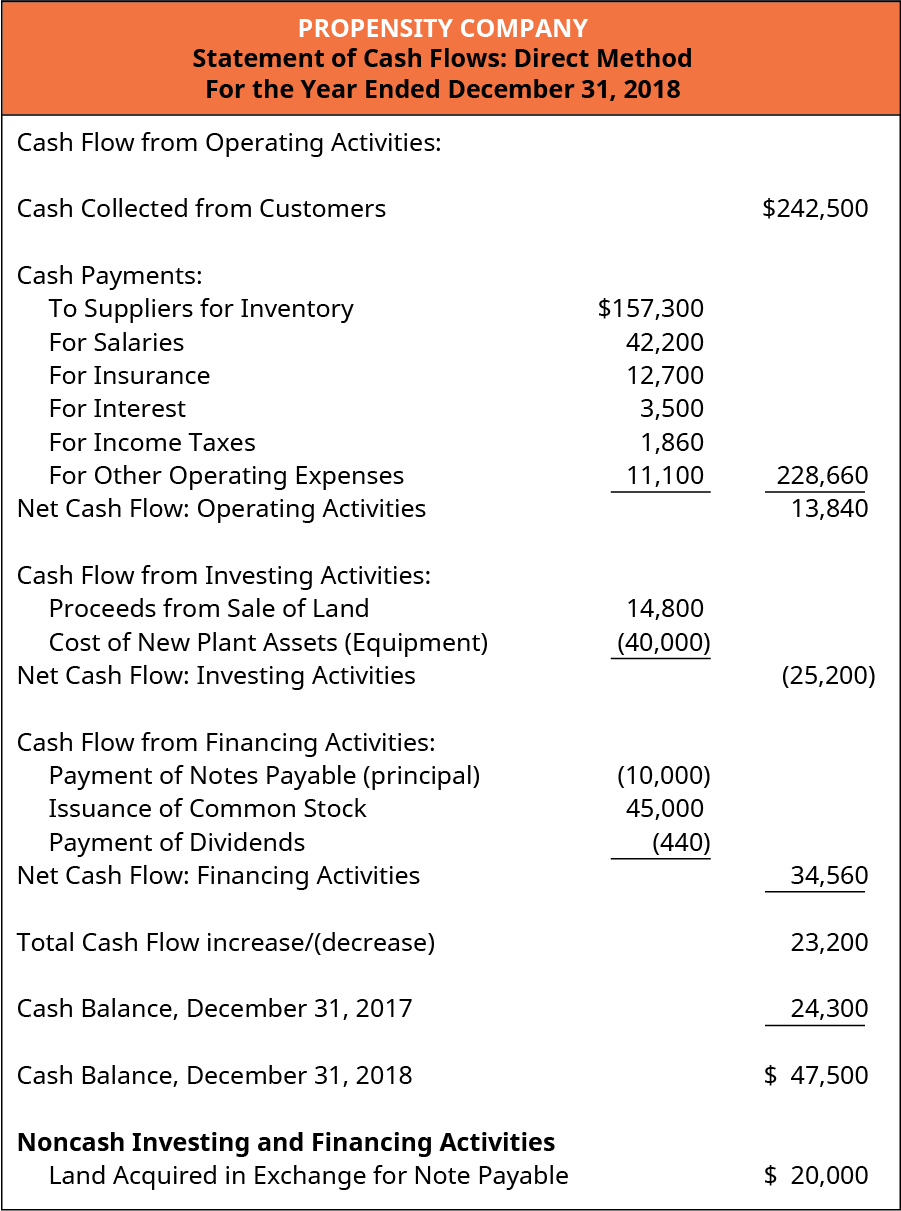As previously mentioned, the net cash flows for all sections of the statement of cash flows are identical when using the direct method or the indirect method. The difference is just in the way that net cash flows from operating activities are calculated and presented. The direct approach requires that each item of income and expense be converted from the accrual basis value to the cash basis value for that item. This is accomplished by adjusting the accrual amount for the revenue or expense by any related current operating asset or liability. Revenue and expense items that are not related to those current asset and liability accounts would not need an adjustment.

In the following section, we demonstrate the calculations needed to assess the component pieces of the operating section using the direct approach.

### Cash Collected from Customers

Cash collected from customers is different from the sales revenue that is recorded on the accrual basis financial statements. To reconcile the amount of sales revenue reported on the income statement to the cash collected from sales, calculate the maximum amount of cash that could have been collected this period (potential cash collected) by combining (a) the amount that was due from customers on the first day of the period (beginning accounts receivable) and (b) total sales revenue recorded this period. If there were no outstanding accounts receivable balance at the end of the period, then one could reasonably assume that this total was collected in full during this period. Thus, the amount collected for sales can be determined by subtracting the ending accounts receivable balance from the total potential cash that could have been collected.### Cash Paid to Suppliers for Inventory

Cash paid for inventory is different from the cost of goods sold that is recorded on the accrual basis financial statements. To reconcile the amount of cost of goods sold reported on the income statement to the cash paid for inventory, it is necessary to perform two calculations. The first part of the calculation determines how much inventory was purchased, and the second part of the calculation determines how much of those purchases were paid for during the current period.

First, calculate the maximum amount of inventory that was available for sale this period by combining (a) the amount of inventory that was on hand on the last day of the period (ending inventory) and (b) total cost of goods sold recorded this period. If there were no inventory balance at the beginning of the period, then one could reasonably assume that this total was purchased entirely during the current period. Thus, the amount of inventory purchased this period can be determined by subtracting the beginning inventory balance from the total goods (inventory) available for sale.

Second, calculate the maximum amount of cash that could have been paid for inventory this period (total obligation to pay inventory costs) by combining (a) the amount that was due to suppliers on the first day of the period (beginning accounts payable) and (b) total inventory purchases this period, from the first inventory calculation. If there were no outstanding accounts payable balance at the end of the period, then one could reasonably assume that this total was paid in full during this current period. Thus, the amount paid for inventory can be determined by subtracting the ending accounts payable balance from the total obligation to pay inventory costs that could have been paid. The final number of the second calculation is the actual cash paid for inventory.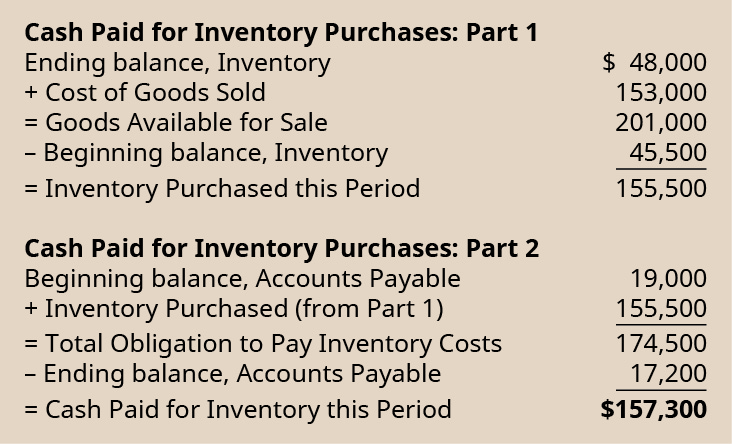### Cash Paid for Salaries

Cash paid for salaries is different from the salaries expense that is recorded on the accrual basis financial statements. To reconcile the amount of salaries expense reported on the income statement to the cash paid for salaries, calculate the maximum amount of cash that could have been paid for salaries this period (total obligation to pay salaries) by combining (a) the amount that was due to employees on the first day of the period (beginning salaries payable) and (b) total salaries expense recorded this period. If there were no outstanding salaries payable balance at the end of the period, then one could reasonably assume that this total was paid in full during this current period. Thus, the amount paid for salaries can be determined by subtracting the ending salaries payable balance from the total obligation to pay salaries that could have been paid.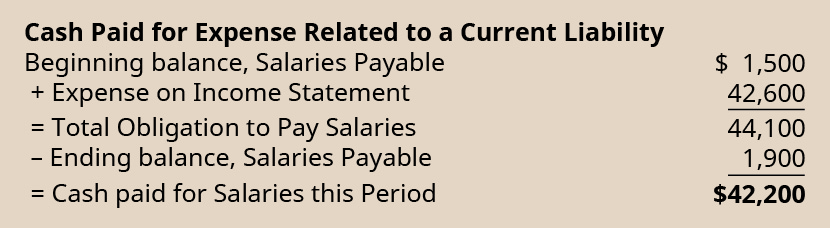### Cash Paid for Insurance

Cash paid for insurance is different from the insurance expense that is recorded on the accrual basis financial statements. To reconcile the amount of insurance expense reported on the income statement to the cash paid for insurance premiums, calculate the maximum amount of cash that could have been paid for insurance this period (total insurance premiums expended) by combining (a) the amount of insurance premiums that were prepaid on the last day of the period (ending prepaid insurance) and (b) total insurance expense recorded this period. If there were no prepaid insurance balance at the beginning of the period, then one could reasonably assume that this total was paid entirely during the current period. Thus, the amount paid for insurance this period can be determined by subtracting the beginning prepaid insurance balance from the total insurance premiums that had been recorded as expended.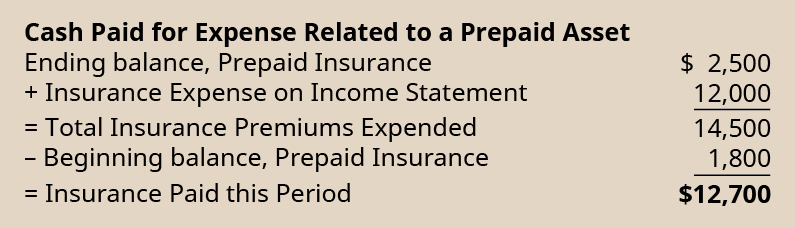### Key Concepts and Summary

• This section included an example of a statement of cash flows, prepared under the direct method, using the continuing example for Propensity Company.
• The direct method of preparing the statement of cash flows is identical to the indirect method except for the cash flows from the operating section.
• To complete the cash flows from operating activities, the direct method directly shows the cash collected from customers from revenue activities and the cash spent on operations, rather than reconciling net income to cash flows from operating activities as done using the indirect method. Calculating the amounts directly collected from revenues and spent on expenditures involves calculating the cash effect of the accrual amounts reported on the income statement.

### Questions

(Figure)Why is using the direct method to prepare the operating section of the statement of cash flows more challenging for accountants than preparing the balance sheet, income statement, and retained earnings statement?

Using the direct method to prepare the operating section requires that revenue and expense items be converted to the cash basis of accounting, since these items are recorded in company records using the accrual basis of accounting. The balance sheet, income statement, and retained earnings statement use the accrual basis balances that are maintained in the company accounting records, and thus can be obtained directly from the adjusted trial balance, without modifications.

### Exercise Set A

(Figure)Use the following excerpts from Algona Company’s financial statements to determine cash received from customers in 2018.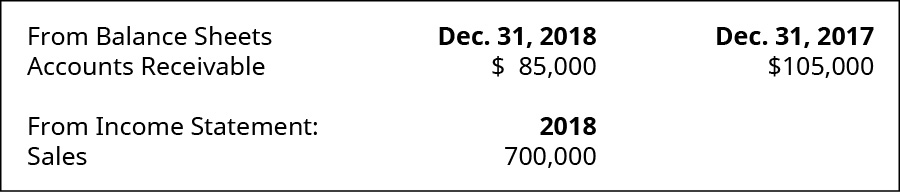(Figure)Use the following excerpts from Huckleberry Company’s financial statements to determine cash paid to suppliers for inventory in 2018.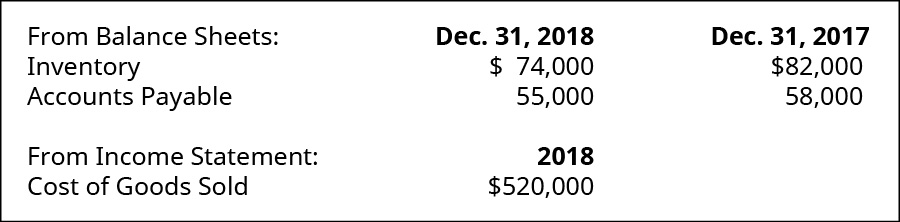### Exercise Set B

(Figure)Use the following excerpts from Brownstone Company’s financial statements to determine cash received from customers in 2018.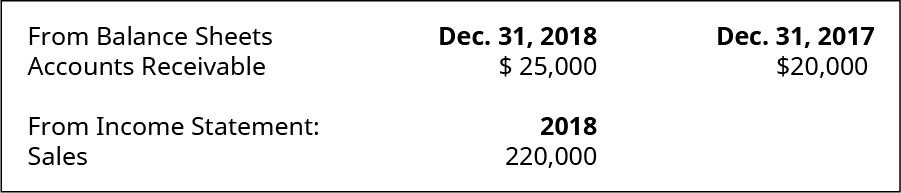(Figure)Use the following excerpts from Jasper Company’s financial statements to determine cash paid to suppliers for inventory in 2018.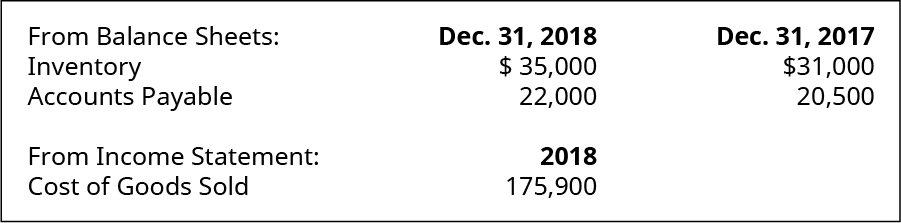### Problem Set A

(Figure)Use the following excerpts from Fromera Company’s financial information to prepare the operating section of the statement of cash flows (direct method) for the year 2018.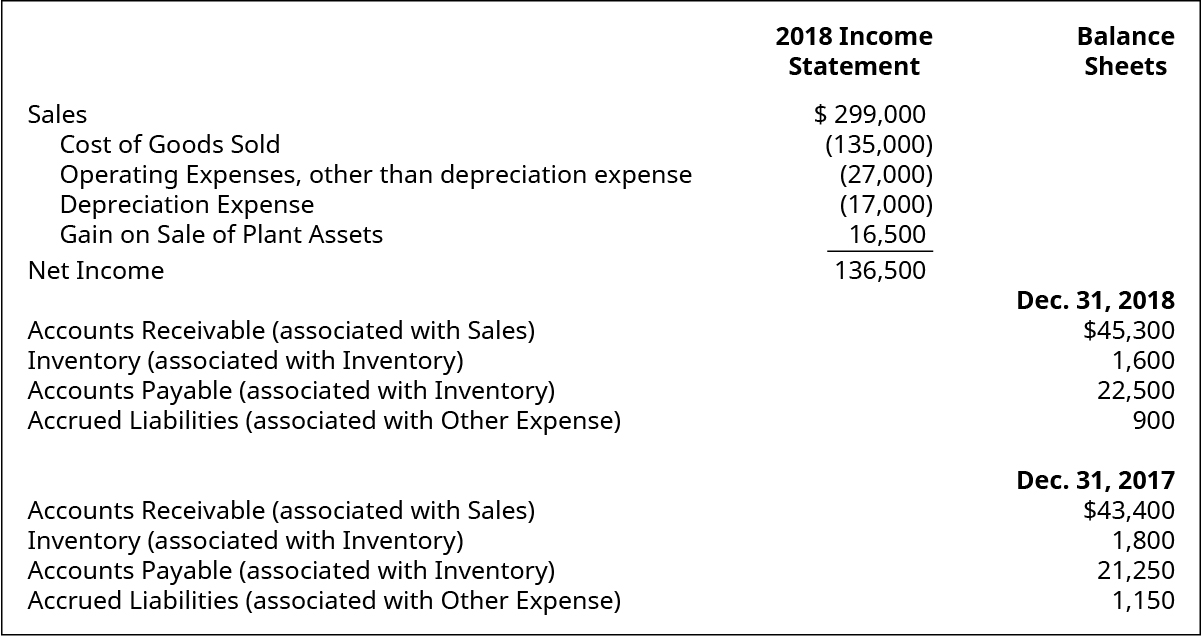(Figure)Use the following excerpts from Victrolia Company’s financial information to prepare a statement of cash flows (direct method) for the year 2018.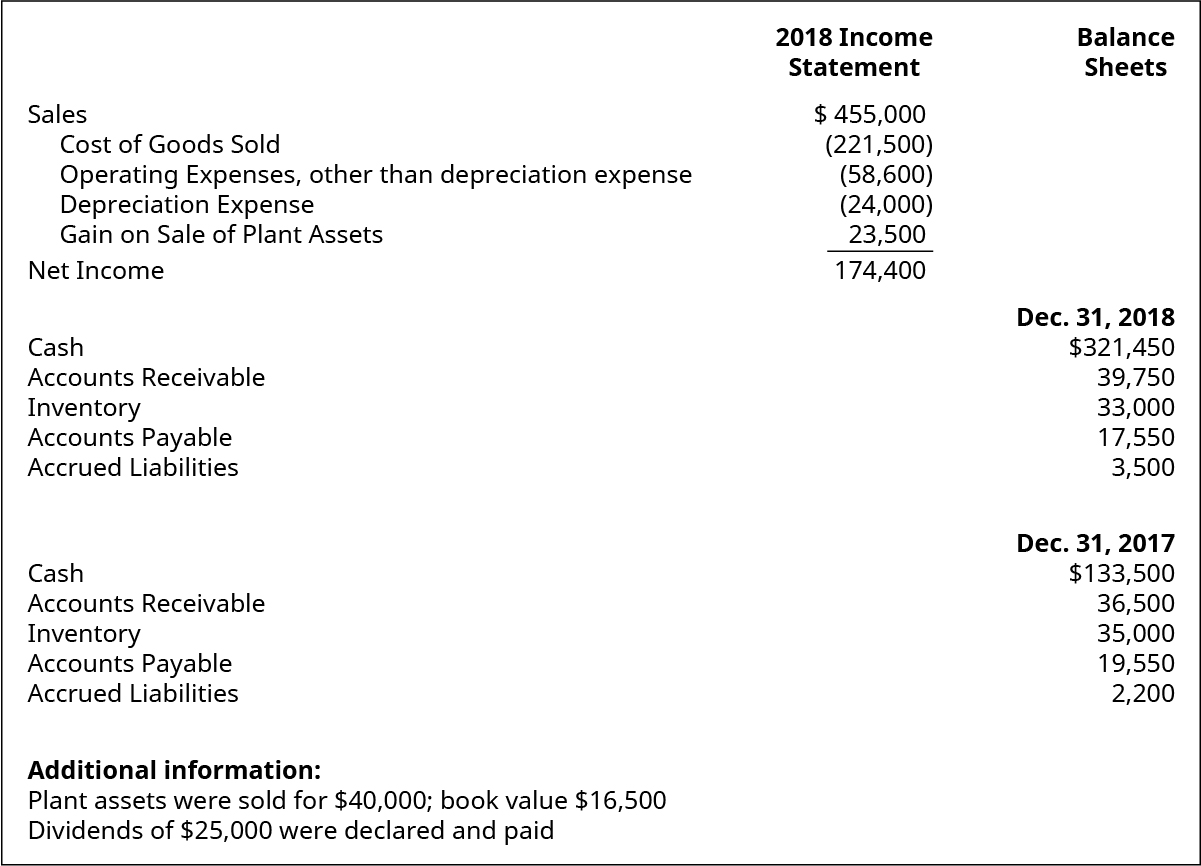(Figure)Use the following cash transactions relating to Lucknow Company to determine the cash flows from operating, using the direct method.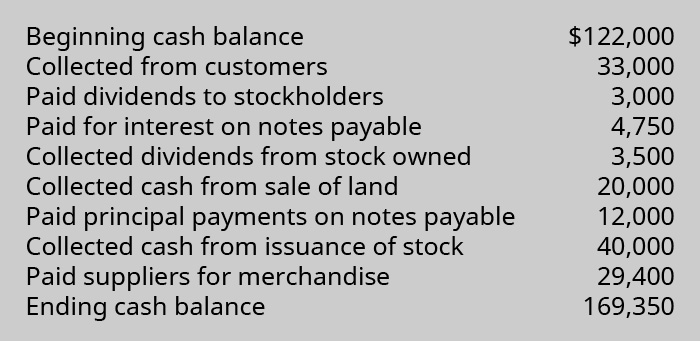### Problem Set B

(Figure)Use the following excerpts from Swansea Company’s financial information to prepare the operating section of the statement of cash flows (direct method) for the year 2018.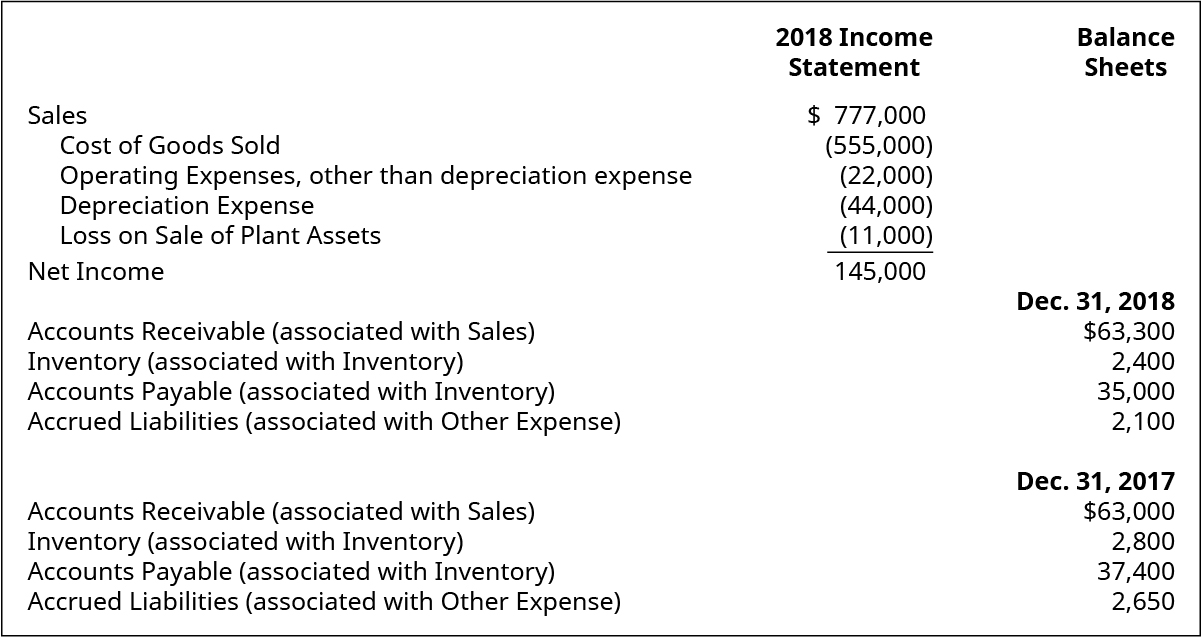(Figure)Use the following excerpts from Swahilia Company’s financial information to prepare a statement of cash flows (direct method) for the year 2018.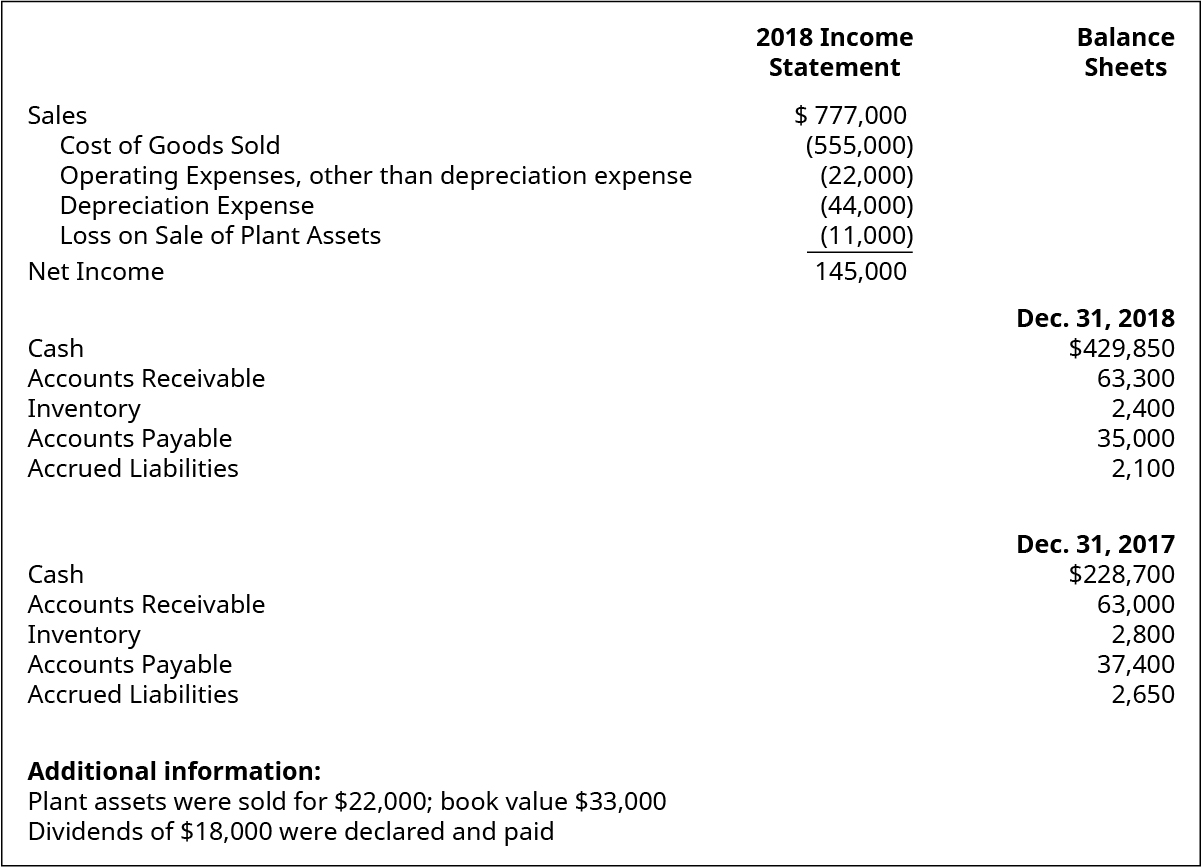(Figure)Use the following cash transactions relating to Warthoff Company to determine the cash flows from operating, using the direct method.